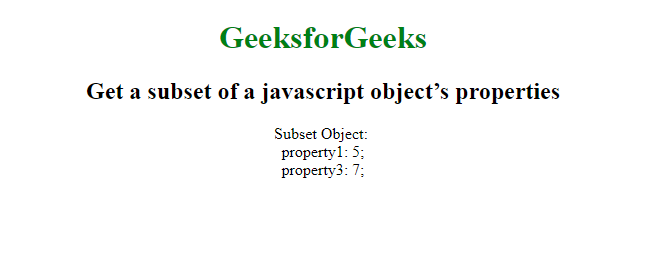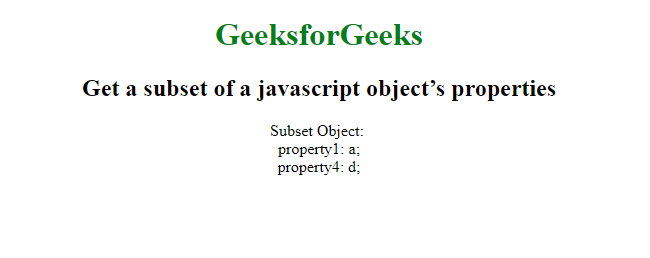# How to get a subset of a javascript object’s properties?

To get the subset of properties of a JavaScript Object, we make use of destructuring and Property Shorthand. The destructuring assignment syntax is a JavaScript expression that makes it possible to unpack values from arrays, or properties from objects, into distinct variables.

Syntex:

`subset = (({a, c}) => ({a, c}))(obj);`

Example1: Get subset of a javascript object’s properties using destructuring assignment.

 ` ` `<``html``> ` ` `  `<``head``> ` `    ``<``title``> ` `      ``Get a subset of a javascript object’s properties ` `  `` ` ` ` ` `  `<``body``> ` `    ``<``center``> ` `        ``<``h1` `style``=``"color:green"``> ` `          ``GeeksforGeeks ` `      `` ` `        ``<``h2``> ` `          ``Get a subset of a javascript object’s properties ` `      `` ` `        ``<``script``> ` `            ``obj = { ` `                ``property1: 5, ` `                ``property2: 6, ` `                ``property3: 7 ` `            ``}; ` `            ``subset = (({ ` `                ``property1, property3 ` `            ``}) => ({ ` `                ``property1, property3 ` `            ``}))(obj); ` ` `  `            ``var output = 'Subset Object: <``br``>'; ` `            ``for (var property in subset) { ` `                ``output += property + ': ' + subset[property] + ';<``br``>'; ` `            ``} ` `            ``document.write(output); ` `        `` ` `    `` ` ` ` ` `  ` `

Output:In ES6 there is a very concise way to do this using destructing. Destructing allows you to easily add on to objects and make subset objects.

Syntax:

```const {c, d, ...partialObject} = object;
const subset = {c, d};```

Example 2: Get subset of a javascript object’s properties using destructuring assignment.

 ` ` `<``html``> ` ` `  `<``head``> ` `    ``<``title``> ` `      ``Get a subset of a javascript object’s properties ` `  `` ` ` ` ` `  `<``body``> ` `    ``<``center``> ` `        ``<``h1` `style``=``"color:green"``> ` `          ``GeeksforGeeks ` `      `` ` `        ``<``h2``> ` `          ``Get a subset of a javascript object’s properties ` `      `` ` `        ``<``script``> ` `            ``const object = { ` `                ``property1: 'a', ` `                ``property2: 'b', ` `                ``property3: 'c', ` `                ``property4: 'd', ` `            ``} ` ` `  `            ``const { ` `                ``property1, ` `                ``property4, ` `                ``...pObject ` `            ``} ` ` `  `            ``= object; ` `            ``const subset = { ` `                ``property1, ` `                ``property4 ` `            ``} ` ` `  `            ``; ` `            ``var output = 'Subset Object: <``br``>'; ` `            ``for (var property in subset) { ` `                ``output += property + ': ' + ` `                    ``subset[property] + ';<``br``>'; ` `            ``} ` ` `  `            ``document.write(output); ` `        `` ` `    `` ` ` ` ` `  ` `

Output:My Personal Notes arrow_drop_upIf you like GeeksforGeeks and would like to contribute, you can also write an article using contribute.geeksforgeeks.org or mail your article to contribute@geeksforgeeks.org. See your article appearing on the GeeksforGeeks main page and help other Geeks.

Please Improve this article if you find anything incorrect by clicking on the "Improve Article" button below.

Article Tags :

Be the First to upvote.

Please write to us at contribute@geeksforgeeks.org to report any issue with the above content.